# Fraction calculator

The calculator performs basic and advanced operations with fractions, expressions with fractions combined with integers, decimals, and mixed numbers. It also shows detailed step-by-step information about the fraction calculation procedure. Solve problems with two, three, or more fractions and numbers in one expression.

## Result:

### 2/5^2 = 4/25 = 0.16

Spelled result in words is four twenty-fifths.

### How do you solve fractions step by step?

1. Exponentiation: 2/5 ^ 2 = 22/52 = 4/25

In other words - two fifths raised to the power of squared = four twenty-fifths.

#### Rules for expressions with fractions:

Fractions - use the slash “/” between the numerator and denominator, i.e., for five-hundredths, enter 5/100. If you are using mixed numbers, be sure to leave a single space between the whole and fraction part.
The slash separates the numerator (number above a fraction line) and denominator (number below).

Mixed numerals (mixed fractions or mixed numbers) write as non-zero integer separated by one space and fraction i.e., 1 2/3 (having the same sign). An example of a negative mixed fraction: -5 1/2.
Because slash is both signs for fraction line and division, we recommended use colon (:) as the operator of division fractions i.e., 1/2 : 3.

Decimals (decimal numbers) enter with a decimal point . and they are automatically converted to fractions - i.e. 1.45.

The colon : and slash / is the symbol of division. Can be used to divide mixed numbers 1 2/3 : 4 3/8 or can be used for write complex fractions i.e. 1/2 : 1/3.
An asterisk * or × is the symbol for multiplication.
Plus + is addition, minus sign - is subtraction and ()[] is mathematical parentheses.
The exponentiation/power symbol is ^ - for example: (7/8-4/5)^2 = (7/8-4/5)2

#### Examples:

subtracting fractions: 2/3 - 1/2
multiplying fractions: 7/8 * 3/9
dividing Fractions: 1/2 : 3/4
exponentiation of fraction: 3/5^3
fractional exponents: 16 ^ 1/2
adding fractions and mixed numbers: 8/5 + 6 2/7
dividing integer and fraction: 5 ÷ 1/2
complex fractions: 5/8 : 2 2/3
decimal to fraction: 0.625
Fraction to Decimal: 1/4
Fraction to Percent: 1/8 %
comparing fractions: 1/4 2/3
multiplying a fraction by a whole number: 6 * 3/4
square root of a fraction: sqrt(1/16)
reducing or simplifying the fraction (simplification) - dividing the numerator and denominator of a fraction by the same non-zero number - equivalent fraction: 4/22
expression with brackets: 1/3 * (1/2 - 3 3/8)
compound fraction: 3/4 of 5/7
fractions multiple: 2/3 of 3/5
divide to find the quotient: 3/5 ÷ 2/3

The calculator follows well-known rules for order of operations. The most common mnemonics for remembering this order of operations are:
PEMDAS - Parentheses, Exponents, Multiplication, Division, Addition, Subtraction.
BEDMAS - Brackets, Exponents, Division, Multiplication, Addition, Subtraction
BODMAS - Brackets, Of or Order, Division, Multiplication, Addition, Subtraction.
GEMDAS - Grouping Symbols - brackets (){}, Exponents, Multiplication, Division, Addition, Subtraction.
Be careful, always do multiplication and division before addition and subtraction. Some operators (+ and -) and (* and /) has the same priority and then must evaluate from left to right.

## Fractions in word problems:

• Hamster cage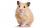Ryan keeps his hamster cage on his dresser. The area of the top of Ryan's dresser is 1 2\3 as large as the area of the bottom of his hamster cage. The area of the dresser top is 960 square inches. How many square inches of his dresser top are not covered
• Cutting square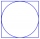From a square with a side of 30 cm, we cut the circle with the highest possible diameter. How many percents of the square content is this circle?
• Square metal sheetWe cut out four squares of 300 mm side from a square sheet metal plate with a side of 0,7 m. Express the fraction and the percentage of waste from the square metal sheet.
• Two brothersThe two brothers were to be divided according to the will of land at an area of 1ha 86a 30m2 in a ratio of 5:4. How many will everyone get?
• The tankThe tank is full up to 4/5 of the total height and contains 240 hl of water. The area of the base is 6 square meters. What is the height of the tank?
• Profit growthThe profit of a company increased by 25% during the year 1992, increased by 40% during the year 1993, decreased by 20% in 1994 and increased by 10% during the year 1995. Find the average growth in the profit level over the four years periods?
• Three shapes1/5 of a circle is shaded. The ratio of area if square to the sum of area of rectangle and that of the circle is 1:2. 60% of the square is shaded and 1/3 of the rectangle is shaded. What is the ratio of the area of circle to that of the rectangle?
• Cube cutIn the ABCDA'B'C'D'cube, it is guided by the edge of the CC' a plane witch dividing the cube into two perpendicular four-sided and triangular prisms, whose volumes are 3:2. Determine in which ratio the edge AB is divided by this plane.
• ConeCircular cone of height 15 cm and volume 5699 cm3 is at one-third of the height (measured from the bottom) cut by a plane parallel to the base. Calculate the radius and circumference of the circular cut.
• Martha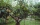Martha likes to walk in the park, the park is square, 7/10 mi on each side. One morning Martha walked around the entire park 3 1/2 times before stopping to rest. How far had she walked?
• Mr. Happy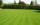Mr. Happy planted 36.6 meters square gardens grass; It's a third of the garden more than half of the garden. What is square area of this garden?
• Simplest form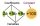Find the simplest form of the following expression: 3 to the 2nd power - 1/4 to the 2nd power.
• Square sides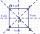If we enlarge the side of the square by a third, its circumference will be 20 cm larger than the original. What is the side of the square?